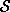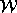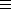## CGAL::Width_3<Traits>

### Definition

Given a set of points={p1,..., pn} in3. The width of, denoted as(), is defined as the minimum distance between two parallel planes of support of conv(); where conv() denotes the convex hull of. The width in direction d, denoted asd(), is the distance between two parallel planes of support of conv(), which are orthogonal to d.

Subject to the applications of the width algorithm, several objects might be interesting:

1. The two parallel planes of support such that the distance between them is as small as possible. These planes are called width-planes in further considerations.
2. The width(), i.e., the distance between the width-planes.
3. The direction dopt such that()=dopt()

Note: There might be several optimal build directions. Hence neither the width-planes nor the direction dopt are unique - only the width is.

#include <CGAL/Width_3.h>

### Requirements

The template parameter Traits is a model for WidthTraits_3. We provide the model Width_default_traits_3<Kernel> based on a three-dimensional CGAL kernel.

### Types

 Width_3::Traits traits class.
 typedef typename Traits::Point_3 Point_3; point type. typedef typename Traits::Plane_3 Plane_3; plane type. typedef typename Traits::Vector_3 Vector_3; vector type. typedef typename Traits::RT RT; algebraic ring type. typedef typename Traits::ChullTraits ChullTraits; traits class for the 3D convex hull algorithm.

### Creation

template < class InputIterator >
Width_3<Traits> width ( InputIterator first, InputIterator beyond);
creates a variable width initialized to the width of- withbeing the set of points in the range [first,beyond).
 Requirement: The value type of InputIterator is Point_3.

template < class Polyhedron >
Width_3<Traits> width ( Polyhedron& P);
creates a variable width initialized to the width of the polyhedron P. Note that the vertex point coordinates are altered!
 Precondition: P is a convex polyhedron.
 Requirement: Polyhedron is a CGAL::Polyhedron_3 with facets supporting plane equations where Polyhedron::Point_3Point_3 and Polyhedron::Plane_3Plane_3.

### Access Functions

 void width.get_squared_width ( RT& width_num, RT& width_denom) returns the squared width. For the reason of exact computation not the width itself is stored, but the squared width as a fraction: The numerator in width_num and the denominator in width_denom. The width of the point setis sqrt((width_num)/(width_denom)). void width.get_width_planes ( Plane_3& e1, Plane_3& e2) The planes e1 and e2 are the two parallel supporting planes, which distance is minimal (among all such planes). void width.get_width_coefficients ( RT& A, RT& B, RT& C, RT& D, RT& K) The returned coefficients A,B,C,D,K have the property that width-plane e1 is given by the equation Ax+By+Cz+D=0 and width-plane e2 by Ax+By+Cz+K=0. Vector_3 width.get_build_direction () returns a direction dopt such that the width-planes e1 and e2 are perpendicular to dopt. The width of the point set is minimal in this direction. void width.get_all_build_directions ( std::vector& dir) All the build directions are stored in the vector dir. It might happen that a certain body has several different build directions, but it is also possible to have only one build direction. int width.get_number_of_optimal_solutions () returns the number of optimal solutions, i.e., the number of optimal build directions.

### Implementation

Since the width of the point setand the width of the convex hull of(conv()) is the same, the algorithm uses the 3D convex hull algorithm CGAL provides.

The width-algorithm is not incremental and therefore inserting and erasing points cause not an `automatic' update of the width. Instead you have to run the width-algorithm again even if the point set is extended by only one new point.advancedLarge Numbers.

Because there is no need for dividing values during the algorithm, the numbers can get really huge (all the computations are made using a lot of multiplications). Therefore it is strongly recommended to use a number type that can handle numbers of arbitrary length (e.g., leda_integer in combination with the homogeneous representation of the points). But these large numbers have a disadvantage: Operations on them are slower as greater the number gets. Therefore it is possible to shorten the numbers by using the compiler flag -DSIMPLIFY. For using this option it is required that the underlying number type provides the `modulo' operation.

Information Output during the Computations.

If during the algorithm the program should output some information (e.g., during the debugging phase) you can turn on the output information by giving the compiler flag DEBUG. In the file width_assertions.h you can turn on/off the output of some functions and additional informations by changing the defined values from 0 (no output) to 1 (output available). But then it is required that the <<-operator has to been overloaded for Point_3, Plane_3, Vector_3 and RT.advanced### Example

```File: examples/Width_3/width_simplex.cpp
```
```#include <CGAL/Homogeneous.h>
#include <CGAL/Width_default_traits_3.h>
#include <CGAL/Width_3.h>
#include <iostream>
#include <vector>

#if defined(CGAL_USE_GMP)
#include <CGAL/Gmpz.h>
typedef CGAL::Gmpz                           RT;
#elif defined (CGAL_USE_LEDA)
#include <CGAL/leda_integer.h>
typedef leda_integer                          RT;
#else
#include <CGAL/MP_Float.h>
typedef CGAL::MP_Float                        RT;
#endif

typedef CGAL::Homogeneous<RT>                 Kernel;
typedef Kernel::Point_3                       Point_3;
typedef Kernel::Plane_3                       Plane_3;
typedef CGAL::Width_default_traits_3<Kernel>  Width_traits;
typedef CGAL::Width_3<Width_traits>           Width;

int main() {
// Create a simplex using homogeneous integer coordinates
std::vector<Point_3> points;
points.push_back( Point_3(2,0,0,1));
points.push_back( Point_3(0,1,0,1));
points.push_back( Point_3(0,0,1,1));
points.push_back( Point_3(0,0,0,1));

// Compute width of simplex
Width simplex( points.begin(), points.end());

// Output of squared width, width-planes, and optimal direction
RT wnum, wdenom;
simplex.get_squared_width( wnum, wdenom);
std::cout << "Squared Width: " << wnum << "/" << wdenom << std::endl;

std::cout << "Direction: " << simplex.get_build_direction() << std::endl;

Plane_3  e1, e2;
std::cout << "Planes: E1: " << e1 << ".  E2: " << e2 <<std::endl;

std::cout << "Number of optimal solutions: "
<< simplex.get_number_of_optimal_solutions() << std::endl;
return(0);
}
```